# A simple introduction to Activation Functions

According to Wikipedia , “In artificial neural network, activation function of a node defines the output of that node given an input or set of inputs”. In a neural network there are three types of layers namely,

Input layer: This layer accepts and passes the inputs to the hidden layer.

Hidden layer: This layer/layers performs numerical computations on the inputs and passes it to the output layer.

Output layer: This layer gives the output of the neural network.

Activation functions are applied in the hidden and output layers. Neurons compute the weighted sum along with bias, activation function inputs this value and decides whether a neuron should be activated or not.

All hidden layers usually use same type of activation function. The type of activation function in the output layer is decided on the type of prediction being made by the model.

There are many types of activation functions, we will discuss the below

1. Linear
2. Sigmoid
3. Tanh
4. Softmax
5. Rectified Linear Unit (ReLU)

## Linear

A linear transform is an identity function. In simple terms identity function is a function which returns an output value which is same as input value. Linear transform is used in the input layer of the neural networks.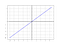Fig 1: Linear activation function

Range: (-infinity, infinity)

## Sigmoid

A sigmoid function reduces the input value to a value between 0 and 1. This function is used mostly in output layer in classification problems where the output should be between 0 and 1.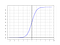Fig 2: Sigmoid activation function

One of the disadvantages of the sigmoid function is if the value of x is very large or very small then the slope becomes close to 0. This can slow down gradient descent from reaching convergence.

## Tanh

tanh function ranges between (-1,1), it can handle negative values. This is similar to sigmoid function with the S curve shifted to origin.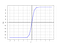Fig 3: Tanh activation function

tanh can also slow down gradient descent with high or low values of x.

## Rectified Linear Unit ( ReLU)

ReLU activates the neuron if the value computed by neuron is greater than a certain value. ReLU is a is a default choice for the hidden layers.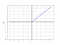Fig 4: ReLU activation funtion

ReLU is advantageous over sigmoid and tanh activation function as it solves the vanishing gradients problem. Hence, gradient descent works well. There is a variant of ReLU called Leaky ReLU where the value of the function is not 0 when x <0. But it is a small negative slope.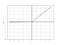Fig 5: Leaky Relu activation function

## Softmax

Usually used in the output layer of a neural network ,softmax function allows the output layer to produce probability distribution over mutually exclusive output classes. Below is a sample input and output of the function.

The output shows three probabilities with the instance being classified to class 1 with probability 0.66

Thank you for reading !

References

I am a data scientist. I write blogs related to machine learning.

## More from Nanda

I am a data scientist. I write blogs related to machine learning.